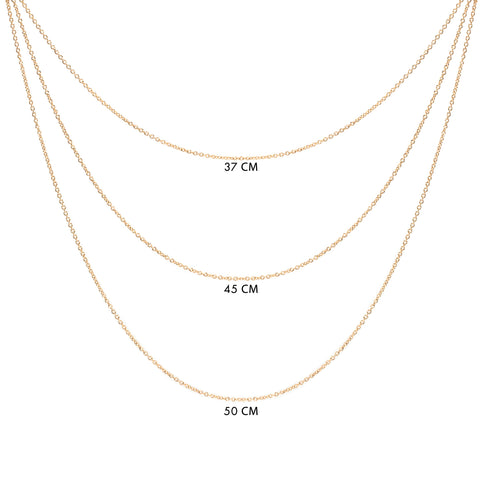# CHAINS LENGTH32+2.5+2.5=37/ 1.26'+0.09'+0.09'=1.45'
40+2.5+2.5= 45/ 1.57'+0.09'+0.09'=1.77'
45+2.5+2.5=50/ 1.77'+ 0.09'+0.09'= 1.97'